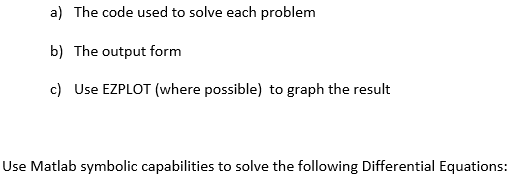# How graph second order differential equations with Matlab?

173 ビュー (過去 30 日間)
jake thompson 2018 年 1 月 18 日
Commented: Rena Berman 2019 年 12 月 12 日
Looking to get some help on how to use matlab to solve the following equation problem 6, all help is appreciated! Thank You! I haven't used matlab in 2 years very rusty, image is reference to original problem statement.4y"-20y'+25y=0
%%PROBLEM 6
clear; syms y(x)
ode = 4*diff(y,x,2) - 20*diff(y,x) + 25*y == 0;
%ySol(x) = dsolve(ode)
%ezplot('ode')
ySol = dsolve(ode, y(0) == 0)
figure
subplot(2,1,1)
ezplot(real(ySol(1)))
subplot(2,1,2)
ezplot(imag(ySol(1)))
ERROR
Warning: Contour not rendered for constant ZData
> In contour (line 52)
In ezplot>ezimplicit (line 315)
In ezplot (line 153)
In sym/ezplot (line 61)

#### 1 件のコメント

Rena Berman 2019 年 12 月 12 日

サインイン to comment.

### 採用された回答

Star Strider 2018 年 1 月 18 日
The problem is that you need to define an initial condition for the first derivative.
Try this:
syms y(x)
Dy = diff(y);
D2y = diff(y,2);
ode = 4*D2y - 20*Dy + 25*y == 0;
ySol = dsolve(ode, y(0) == 0, Dy(0) == 1) % Define The Initial Condition For ‘Dy(0)’ To Be ‘Some Value’
figure
ezplot(ySol)

#### 3 件のコメント

jake thompson 2018 年 1 月 18 日
Thank you again!
Star Strider 2018 年 1 月 18 日
As always, my pleasure!
Hamid Ghassri 2019 年 7 月 8 日
how can you set a range for x

サインイン to comment.